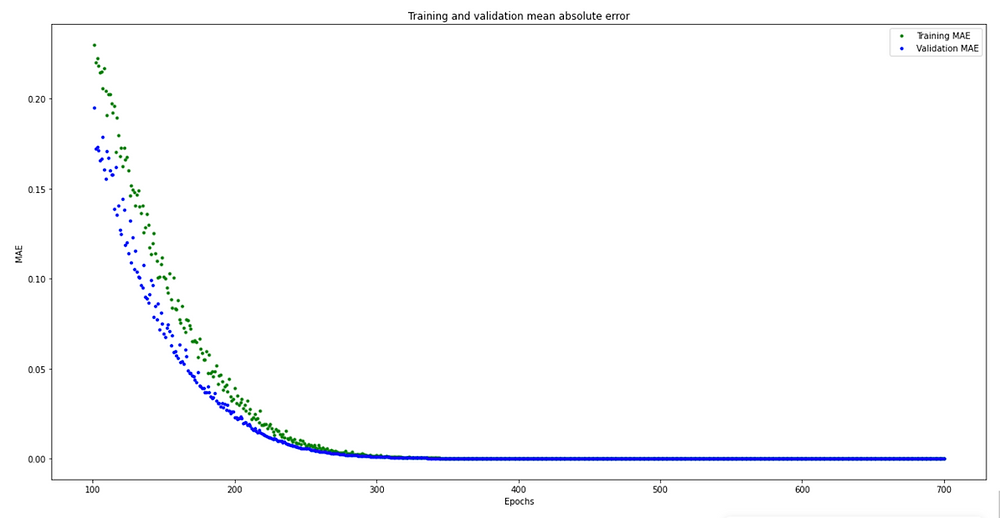Search
• KJ Ha

# ML4PCOMP Assignment 2

Since I've experienced some difficulties with the magic wand example even after modifying LSM9DS1.cpp file and I remembered getting the accurate results (e.g. getting the ring shape right) is one of the main issues of the example, I decided to build my own model using the tinyml workshop as a reference.

I followed the steps found on Get started with machine learning on Arduino tutorial. I first collected data for two gestures, 'circle' and 'w' using IMU Capture.ino and created separate csv file for each gesture.

Then I moved to Google Colab to create the model based on my own datasets. After I realized that the loss of the model is not as stable as I expected (it might be due to initial flaws in datasets), I tried to optimize the model by hyperparameter tuning. I've tried ReLu, Leaky ReLu, and Sigmoid for loss functions and interestingly among those functions, a mixture of Sigmoid and ReLu functions gave me the most stable result in terms of the loss.

One of the major differences between the ReLu and Sigmoid functions is the one regards to productivity and generally speaking, the ReLu function is considered better, at least preferred, for that reason. However, in this specific case, partially because the target model is relatively light, this transition did not severely affect the speed of training.

Initial code

```
# build the model and train it
model = tf.keras.Sequential()
model.add(tf.keras.layers.Dense(NUM_GESTURES, activation='softmax')) # softmax is used, because we only expect one gesture to occur per input
model.compile(optimizer='rmsprop', loss='mse', metrics=['mae'])
history = model.fit(inputs_train, outputs_train, epochs=600, batch_size=1, validation_data=(inputs_validate, outputs_validate))

```

Modified

```
# build the model and train it
model = tf.keras.Sequential()
model.add(tf.keras.layers.Dense(NUM_GESTURES, activation='softmax')) # softmax is used, because we only expect one gesture to occur per input
model.compile(optimizer='rmsprop', loss='mse', metrics=['mae'])
history = model.fit(inputs_train, outputs_train, epochs=700, batch_size=1, validation_data=(inputs_validate, outputs_validate))

```

### The loss graph

Using ReLu function onlyUsing a mixture of ReLu and Sigmoid functions### The loss graph skipping a bit of the start

Using ReLu function onlyUsing a mixture of ReLu and Sigmoid functions### The mean absolute error graph

Using ReLu function onlyUsing a mixture of ReLu and Sigmoid functionsI'm well aware that there might be errors in the initial data I collected because of Arduino BLE not being the most robust device to capture gestures or of my lack of precision in capturing those gestures. Thus hyperparameter tuning might be just a mere torturing on the machine or data itself rather than improvement for the model. However, I believe that it was worth testing out different loss functions and values to see different results in training. And I was satisfied with the results I got using IMU Classifier.ino.

### Recent Posts

See All

#### ML4PCOMP Assignment 4

I have two separate ideas for the final project. Similar to the project I did for Intro to Pcomp class, I would like to build a simple watering device using a teachable machine algorithm. The device w